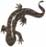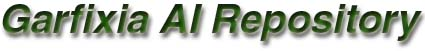Last updated:

Sparse Distributed Memory is a theory and working model of human long-term-memory. It is completely (and only) described in Pentti Kanerva's book "Sparse Distributed Memory". It provides a fascinating insight into the (possible) workings of human memory and it does a good job as an AI tecnhique as well. Possible uses:

• Best-Match: From a set of patterns and a test-pattern that is like, but not equal to, one of the patterns in the set, find this best-matching pattern.
• Associations: Associate one pattern with another.
• Sequences: Associate one pattern with the next. Use the memory to retrieve sequences of patterns.
• Prediction: Predict what the next pattern will be given a sequence of previous patterns.

The model can be used to store patterns with many dimensions, in a compact way. However, I think there are neural networks that store the data even much more compactly, so if this is a concern, don't just choose SDM right away.

What was implemented:
• A set of hard-locations whose addresses are randomized.
• The most suitable number-of-hard-locations can be calculated automatically, based on the number of words you intend to store and the word's bitcount.
• The access-radius can be calculated automatically.
• Writing address-value pairs, with a special case where the address equals the value (a content-addressable memory).
• Reading the archetype (or mean) value from memory given a certain address. The process can also be performed iteratively (in a best-match case).
• Pooling multiple words in a hard-location.
• Some query functions to find out the current state of the memory; notably: capacity and overload.
• I created two sample applications: one to demonstrate the best-match use of the memory, and one that implements a k-fold predication mechanism (k=3).
Remarks
• Storing words in a storage location. Kanerva suggests that multiple words are stored in a storage location by keeping one counter for each of the bits in the word. Then he suggests adding 1 for each 1 that is added and subtracting 1 for each 0 that is added (page75). I have a problem with this. These words are mainly used to determine an archetype word at a time when some address is read. The archetype is the mean of all words that can be read from some address. If a storage location contains multiple words it should bring in more weight than a storage location with fewer words. However, with the suggested representation this weight is not represented correctly. Take for example one location with the single word 1111 (represented as "+1+1+1+1". It weights in for 100% in the archetype calculation. Take on the other hand a location with the words 1000, 1000, 0100, 0100: It's representation is " 0 0 0 0". Even though the location contains more words, it weights in less then the location with only one word. You may consider multiplying the first representation by 1 and the second by 4 (the number of words), but this will not improve matters since 4 * 0 = 0. The mean value would be "+1+1+1+1"/5 = " 0 0 0 0", which is incorrect. Therefore I chose a storage representation with counters, adding 1 for each 1 and adding 0 for each 0. In the example this yields "+1+1+1+1" for the first location and "+2+2 0 0" for the second location. The mean value is "+3+3+1+1" / 5 = "+1+1 0 0". When I was a good deal finished, I tried Kanerva's method again to see how much they differed. I was shocked to see my results corrupted. I may be wrong, but if I'm right this is important!
• Two distinct uses of memory. The contents of memory is treated in chapters 7 and 8 of Kanerva's book. Chapter 7 deals with a Best-Match system (given a value, return the best archetype); chapter 8 deals with a Sequence system (given a situation at time t, return the situation at time t+1). Since Kanerva never explicitly talks about two possible uses of his memory, I assumed that these two systems were integrated into a single memory system. So my first implementation contained two data pools in each storage location: one for the Best-Match system and one for the Sequence system. I then added code so that when writing a word to the system, it would also be written to the transition field of the storage locations in the previous time-step. Somehow this started to seem like a lot of work, while I could find no such thing explicit in Kanerva's book. In the chapter on Sequences Kanerva simply tells us to write the address of time-2 at time-1. Studying other SDM implementations on the Internet (mentioned below) it dawned on me that SDM is used in two different applications. In the first, Best-Match, way, words are written and are read iteratively, to find the best-matching prototype. The address written to and the value written are the same word. In the second application, Sequencer, words are written as well, but the address is different from the value written. When an address is read, it is not read iteratively, since there is no use for this.
• Counter overflow. It didn't become clear to me what should happen when one of the counters of a storage location overflows. At page 75 he suggests that the counter of an overflowing bit does not change when it overflows. This may mean that the other counters can still be updated, but that seems like a bad idea. My implementation: if n words were written to a location and this is the max, a new word written to that location is ignored.
• Prescriptions. Kanerva's book sometimes lacks prescriptions on how to do things. First, he does not mention how many hard-locations you should use in a well-built memory (given the number of words you intend to feed it). Second, he doesn't mention directly what a suitable access-radius is given the number of hard-locations. Guessing these numbers can be a lot of work, and it's my opinion this is not the application-programmers task. So I set out to calculate suitable numbers myself.
• Number of hard-locations. To calculate the right number of hard-locations, I chose the formulas on page 74 of the book. I used them to calculate the number of hard-locations based on the capacity. I calculated 1/H(n), which I called the capacity fraction. I multiplied this by the requested maximum capacity (the expected number of words to be stored) and multiplied this again by 10 so that the memory would not be completely full once all words are added. Applying the formula on Kanerva's numbers (n=1000, 10,000 words to be stored) yielded a number of 1,022,620 hard-locations. About the number Kanerva picked.
• Access-radius. To calculate the most suitable access-radius I chose to calculate the mean nearest-neighbour of two hard-locations. This would be the minimally suitable access-radius. In order to create a reasonable amount of overlap between the access-circles, I pretended that there were 10 times less hard-locations and fed this into the function. The results are satisfying. Using Kanerva's input (n=1000, N=1000000) yielded an access-radius of 437. A number between 425 (nearest neighbour, or 0.000001 of the space) and 451 (a rather random number he works with, or 0.001 of the space).
Sample use (some helper functions were left out)
```#define WIDTH 10
#define HEIGHT 10
#define PATTERN_COUNT 5
#define WORD_LENGTH WIDTH*HEIGHT
#define NUMBER_OF_EXPOSURES 10
#define WORD_COUNT PATTERN_COUNT*NUMBER_OF_EXPOSURES

CSDMModel Model(WORD_LENGTH, WORD_COUNT);
CBitPattern BitPattern(WORD_LENGTH), ResultPattern(WORD_LENGTH);
bool Found;

// write all patterns several times, each with a small distortion
for (int i=0; i < NUMBER_OF_EXPOSURES; i++)
{
for (int PatternIndex=0; PatternIndex < PATTERN_COUNT; PatternIndex++)
{
// create a bitpattern
StringToBitPattern(Patterns[PatternIndex], BitPattern);

// distort the pattern 10%
Distort(BitPattern, 0.1f);

// store the bitpattern
Model.Write(BitPattern);
}
}

for (int PatternIndex=0; PatternIndex < PATTERN_COUNT; PatternIndex++)
{
// get a bitpattern
StringToBitPattern(Patterns[PatternIndex], BitPattern);

Distort(BitPattern, 0.1f);

printf("Input:\n");
PrintPattern(BitPattern);

printf("Output:\n");
if (!Found)
else
{
// print it
PrintPattern(ResultPattern);
}
printf("\n");
}

// print some info
printf("Bitcount:\t%d\n", Model.GetBitCount());